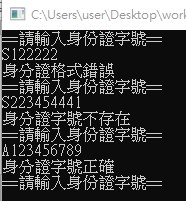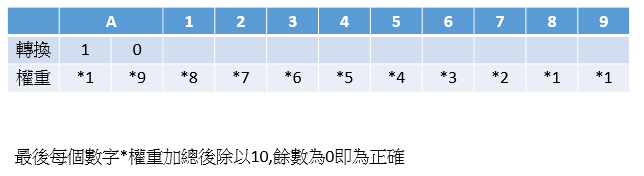DAY 19
0
Modern Web

# 什麼是正規表達式Regular Expression?

Regular Expression指的是用來比對字串是否符合指定格式的表示式。所屬命名空間為System.Text.RegularExpressions(就是你要記得寫這一串呼叫或是在using寫進去XD)。屬於Regex類別。

<<狀況劇>>

07-6511111
(07)651-1111
(07)6511111
6511111
0932111111
+88611111111

# Regular Expression寫法

MSDN裡Regex的建構函式有五個，最常使用的有兩個

Regex regex = new Regex(string pattern);
Regex regex = new Regex(string pattern, RegexOptions options);

pattern是你的字串規則
RegexOptions options是字串比對選項，是一個列舉型別，選項部分請參考MSDN說明:RegexOptions Enum

# Regular Expression語法

• 字元:

a : 輸入a代表這個位置要出現a
. : 萬用字元：比對除 \n 以外的任何單一字元
\ : 逸出字元前方要加斜線

寫法 說明 範例
. 含有任意字元的字串 "123yo","你好"
a. a 後面接一個任意字元的字串 "apple","a你好"
• 比較多個字元

[]: 括號內的任何字元
[^]: 不在括號內的任何字元
[-]: 範圍

寫法 說明 範例
[a-z] 含小寫字母的字串 "yo123"
[^a-zA-Z] 不含大小寫字母的字串 "123"
• 位置

^:字串開頭
\$:字串結尾或字串結尾的 \n 之前
\b:比對必須發生在 \w (英數) 和 \W (非英數) 字元之間的界限上

寫法 說明 範例
^yo 開頭是yo的字串 "yo123"
yo\$ 結尾是yo的字串 "123yo"
• 比較次數

*: 出現 0 次以上
+: 出現1次以上
?: 出現0次或1次
{n}: 出現n次
{n,}: 出現至少n次
{n,m}: 出現n~m次
*?:出現 0 次以上，但越少越好
+?: 出現1次以上，但越少越好
??: 出現0次或1次，但越少越好

寫法 說明 範例
* 出現 0 次以上的a "apple"
* 包含一個 a，後面至少 0 個 b "a","ab"
a+ 出現1次以上的a "aa","aab"
a? 出現0次或1次的a "a","b"
a{3} 出現3次的a "aaa"
a{3,} 出現至少3次的a "aaa","aaaaaa"
a{2,3} 出現2或3次的a "aaa","aa"
• 特殊字元

\w: 比對任何文字字元(含數字字母底線)
\W: 比對任何非文字字元
\s: 比對任何泛空白字元，含空白、換行、tab，等同 [ \f\n\r\t\v]
\S: 比對任何非泛空白字元
\d: 比對任何十進位數字
\D: 比對十進位數字以外的任何字元

• 其他

\p{Lu} (\P{Lu}):檢出大寫(非大寫)的字母, 例如 (?-i:\p{Lu}) 可檢出字串中所有大寫字母, 而 (?-i:\P{Lu}) 可檢出所有非大寫 (包括數字、空白等) 的字母。
():用來括住一群字元，把他們視為一個集合，通常用來集合表示多個檢核式。

# 驗證手機電話號碼

`````` while (true)//方便連續測試用
{
Console.WriteLine("請輸入手機電話號碼:");
bool telcheck = Regex.IsMatch(tel, @"^09[0-9]{8}\$");//規則:09開頭，後面接著8個0~9的數字，@是避免跳脫字元
//isMatch回傳布林值T或F
if (telcheck)
{
Console.WriteLine("這個電話號碼格式正確");
}
else
{
Console.WriteLine("電話格式恩丟喔");
}
Console.WriteLine("============");
}
``````

# 驗證身分證字號

(1)英文代號以下表轉換成數字
A = 10 台北市 J = 18 新竹縣 S = 26 高雄縣
B = 11 台中市 K = 19 苗栗縣 T = 27 屏東縣
C = 12 基隆市 L = 20 台中縣 U = 28 花蓮縣
D = 13 台南市 M = 21 南投縣 V = 29 台東縣
E = 14 高雄市 N = 22 彰化縣* W = 32 金門縣
F = 15 台北縣* O = 35 新竹市 X = 30 澎湖縣
G = 16 宜蘭縣 P = 23 雲林縣 Y = 31 陽明山
H = 17 桃園縣 Q = 24 嘉義縣* Z = 33 連江縣
*I = 34 嘉義市 R = 25 台南縣
(2)，而首位數字則是拿來區分性別，男性為1、女性為2，
2.規則說明：
(1)英文轉成的數字, 個位數乘９再加上十位數
(2)各數字從右到左依次乘１、２、３、４．．．．８
(3)求出(1),(2)之和
(4)求出(3)除10後之餘數,用10減該餘數,結果就是檢查碼,若餘數為0，檢查碼就是0

`````` while (true)//方便連續測試用
{
Console.WriteLine("==請輸入身份證字號==");
//以下開始解法一
string checkID = "";
int Esum = 0;
int Nsum = 0;
int count = 0;
bool flag = Regex.IsMatch(typein, @"^[A-Z]{1}[1-2]{1}[0-9]{8}\$");//先判定是否符合一個大寫字母+1或2開頭的1個數字+8個數字
//先用正規運算式判斷是否符合格式
typein = typein.ToUpper();//把字母轉成大寫
if (flag)//如果符合第一層格式
{   //宣告一個陣列放入A~Z相對應數字的順序
string[] country = new string[] { "A","B", "C", "D", "E", "F", "G", "H", "J", "K", "L", "M", "N", "P", "Q", "R", "S", "T", "U", "V", "X", "Y", "W", "Z", "I", "O" };
for (int index = 0; index < country.Length; index++)
{
if (typein.Substring(0, 1) == country[index])
{
index += 10;//A是從10開始編碼,每個英文的碼都跟index差異10,先加回來
Esum = (((index % 10) * 9) + (index / 10));
//英文轉成的數字, 個位數(把數字/10取餘數)乘９再加上十位數
//加上十位數(數字/10,因為是int,後面會直接捨去)
break;
}
}
for (int i = 1; i < 9; i++)
{//從第二個數字開始跑,每個數字*相對應權重
Nsum += (Convert.ToInt32(typein[i].ToString())) * (9 - i);
}
count = 10 - ((Esum + Nsum) % 10);//把上述的總和加起來,取餘數後,10-該餘數為檢查碼,要等於最後一個數字
if (count == Convert.ToInt32(typein.ToString()))//判斷計算總和是不是等於檢查碼
{
checkID = "身分證字號正確";
}
else
{
checkID = "身分證字號不存在";
}
}
else
{
checkID = "身分證格式錯誤";
}
Console.WriteLine(checkID);
}
````````````while (true)//方便連續測試用
{
Console.WriteLine("==請輸入身份證字號==");
//以下開始解法二
bool flag = Regex.IsMatch(typein, @"^[A-Za-z]{1}[1-2]{1}[0-9]{8}\$");
//使用正規運算式判斷是否符合格式
int[] ID = new int;//英文字會轉成2個數字,所以多一個空間存放變11個
int count = 0;
string result = "";
typein = typein.ToUpper();//把英文字轉成大寫
if (flag == true)//如果符合格式就進入運算
{//先把A~Z的對應值存到陣列裡，分別存進第一個跟第二個位置
switch (typein.Substring(0, 1))//取出輸入的第一個字--英文字母作為判斷
{
case "A": (ID, ID) = (1, 0); break;//如果是A,ID就放入1,ID就放入0
case "B": (ID, ID) = (1, 1); break;//以下以此類推
case "C": (ID, ID) = (1, 2); break;
case "D": (ID, ID) = (1, 3); break;
case "E": (ID, ID) = (1, 4); break;
case "F": (ID, ID) = (1, 5); break;
case "G": (ID, ID) = (1, 6); break;
case "H": (ID, ID) = (1, 7); break;
case "I": (ID, ID) = (3, 4); break;
case "J": (ID, ID) = (1, 8); break;
case "K": (ID, ID) = (1, 9); break;
case "L": (ID, ID) = (2, 0); break;
case "M": (ID, ID) = (2, 1); break;
case "N": (ID, ID) = (2, 2); break;
case "O": (ID, ID) = (3, 5); break;
case "P": (ID, ID) = (2, 3); break;
case "Q": (ID, ID) = (2, 4); break;
case "R": (ID, ID) = (2, 5); break;
case "S": (ID, ID) = (2, 6); break;
case "T": (ID, ID) = (2, 7); break;
case "U": (ID, ID) = (2, 8); break;
case "V": (ID, ID) = (2, 9); break;
case "W": (ID, ID) = (3, 2); break;
case "X": (ID, ID) = (3, 0); break;
case "Y": (ID, ID) = (3, 1); break;
case "Z": (ID, ID) = (3, 3); break;
}
for (int i = 2; i < ID.Length; i++)//把英文字後方的數字丟進ID[]裡
{
ID[i] = Convert.ToInt32(typein.Substring(i - 1, 1));
}
for (int j = 1; j < ID.Length - 1; j++)
{
count += ID[j] * (10 - j);//根據公式,ID*9+ID*8......
}
count += ID + ID;//把沒加到的第一個數加回來
if (count % 10 == 0)//餘數是0代表正確
{
result = "身份證正確";
}
else
{
result = "身份證不存在";
}
}
else
{
result = "身份證格式不正確";
}
Console.WriteLine(result);
}
``````

0